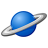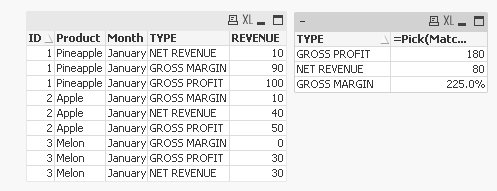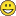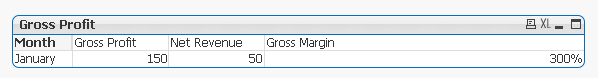QlikView App Dev

Discussion Board for collaboration related to QlikView App Development.

Announcements
QlikWorld 2022, LIVE in Denver CO., May 16-19, 2022. REGISTER NOW TO RECEIVE EARLY BIRD PRICING
cancel
Showing results for
Did you mean:Creator II

Calculating percentages in pivot table

Hi all,

I am doing sales results in qlikview and would like to calculate the total percentage in a line, using pivot table. For each product, I calculate the 'net revenue' , 'gross profit' and divinding gross profit per NET REVENUE we have the GROSS MARGIN. This is only for one product, but there is a problem for obtaining the GROSS MARGIN  for more than one in a pivot table, as I am using SUM() to have total per month.

What I would like to do is even the user selecting one or more product, the GROSS MARGIN would be the result of division (GROSS PROFIT/(NET REVENUE)).

Please see table below with desirable result:

TABLE A:

ID    PRODUCT      GROSS PROFIT          NET REVENUE         GROSS MARGIN               MONTH

1        pineapple         100                                     10                        (100/10) = 1000%             JANUARY

2       apple                     50                                     40                       (50/40)   =       80%            JANUARY

OUTPUT TABLE:

MONTH                GROSS PROFIT          NET REVENUE         GROSS MARGIN

JANUARY                  150                                      50                          (150/50) = 300%

IF I use the sum() it will return the value of 1080% and not the correct (300%).

does anyone knows how to do that?

1 Solution

Accepted SolutionsMVP

This?11 RepliesMVPCreator II
Author

Sorry mate, I have made this way but I was asked to do the way below:

For the first case your answer helped me.The new desirable result is:

JANUARY

GROSS PROFIT                            150

NET REVENUE                               50

GROSS MARGIN                        (150/50) = 300%MVP

Do you have a sample where you are trying this out? May be easy to see how the table and data looks likeChampion III

In the Pivot table Use Month as Dimension and use these below 3 in the Expression tab. It works fine at my end.

Dimension - Month

Expression tab:

1. Sum([Gross Profit])

2. Sum([Net Revenue])

3. (Sum([Gross Profit])/Sum([Net Revenue])) * 100 & '%'     Label As [Gross Margin]

Try this and let us know.Thanks

V.Champion III

Are you looking for something like below:Creator II
Author

Sunny, here is exactly what I am doing in script with another data:

TABLE_1:

ID, Product, GROSS PROFIT, NET REVENUE, Month

1, Pineapple, 100, 10, January

2, Apple, 50, 40, January

3, Melon, 30, 30, January

];

Calculation:

[GROSS PROFIT] - [NET REVENUE] as [GROSS MARGIN]

Resident TABLE_1;

drop table TABLE_1;

NoConcatenate

TMP:

ID,

Product,

Month,

[GROSS PROFIT],

[NET REVENUE],

[GROSS MARGIN]

Resident Calculation;

drop table Calculation;

FINAL:

CrossTable(TYPE, REVENUE, 3)

Resident TMP;

drop Table TMP;

Another question. Do you know how to sort lines, having GROSS MARGIN, GROSS PROFIT and then NET REVENUE?

Thanks.MVP

This?Creator II
Author

Thank you very much, Sunny!

It helped a lot.

Best Regards.Creator II
Author

Hi Sunny,

What if I have the same pivot table with one more dimension:

Dimension

Product

Type

Expression:

Using the same expression in this case gives the same value for every product, even removing the 'TOTAL' out.Community Browser